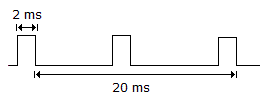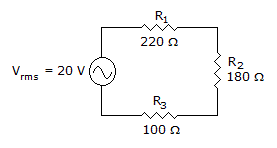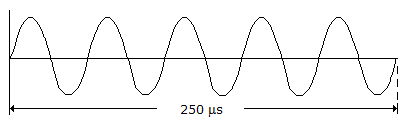# Electronics - Alternating Current and Voltage

### Exercise :: Alternating Current and Voltage - Filling the Blanks

1.The frequency of the repetitive pulse waveform in the given circuit equals ___.

 A. 500 Hz B. 50 Hz C. 100 Hz D. 125 Hz

Answer: Option C

Explanation:

No answer description available for this question. Let us discuss.

2.

Increasing the number of magnetic poles in a basic generator will ___.

 A. increase the generator's output voltage amplitude B. increase the generator's output frequency C. decrease the generator's output voltage amplitude D. decrease the generator's output frequency

Answer: Option B

Explanation:

No answer description available for this question. Let us discuss.

3.The peak voltage across R2 in the given circuit equals _____.

 A. 7.2 V B. 10.18 V C. 8.8 V D. 8 V

Answer: Option B

Explanation:

No answer description available for this question. Let us discuss.

4.The frequency of the sine wave in the given circuit equals ___.

 A. 4 kHz B. 800 Hz C. 20 kHz D. 250 Hz

Answer: Option C

Explanation:

No answer description available for this question. Let us discuss.

5.

Increasing the number of turns in a conductor loop in a basic generator will ___.

 A. increase the generator's output voltage amplitude B. increase the generator's output frequency C. decrease the generator's output voltage amplitude D. decrease the generator's output frequency

Answer: Option A

Explanation:

No answer description available for this question. Let us discuss.

#### Current Affairs 2021

Interview Questions and Answers